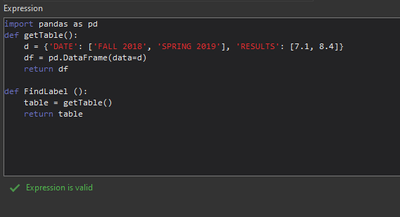# Simple table in Label Expression

210
4
03-21-2023 01:08 PMby
New Contributor II

I want to create simple tables in my labels. I thought that using python and pandas dataframe might work, but although the expression verifies as valid, no labels appear on my map. Is there a different way to accomplish this either in Python or a different language? I am using ArcPro 3.1. ThanksTags (2)
1 Solution

Accepted SolutionsbyMVP Regular Contributor

Labels only show strings, s you have to return a string.

I can' test right now, maybe it works with the DataFrame method:

``````def FindLabel():
table = getTable()
return table.to_string()``````

If it doesn't, you can try constructing it yourself (untested):

``````def FindLabel():
d = {"DATE": ["Fall 2018", "Spring 2019"], "RESULTS": [7.1, 8.4]}
# get the column names and the max row count
columns = k in d.keys()
num_rows = max([len(d[c]) for c in columns])
rows = [
"\t".join([f"<BOL>{c}</BOL>" for c in columns]),
]
# append the values
for r in range(num_rows):
row = []
for c in columns:
# get the value of current r and c, or default empty string
try:
row.append(str(d[c][r]))
except IndexError:
row.append(" ")
rows.append("\t".join(row))
# concatenate the rows with line breaks
return "\n".join(rows)
``````

Have a great day!
Johannes
4 RepliesbyMVP Regular Contributor

Labels only show strings, s you have to return a string.

I can' test right now, maybe it works with the DataFrame method:

``````def FindLabel():
table = getTable()
return table.to_string()``````

If it doesn't, you can try constructing it yourself (untested):

``````def FindLabel():
d = {"DATE": ["Fall 2018", "Spring 2019"], "RESULTS": [7.1, 8.4]}
# get the column names and the max row count
columns = k in d.keys()
num_rows = max([len(d[c]) for c in columns])
rows = [
"\t".join([f"<BOL>{c}</BOL>" for c in columns]),
]
# append the values
for r in range(num_rows):
row = []
for c in columns:
# get the value of current r and c, or default empty string
try:
row.append(str(d[c][r]))
except IndexError:
row.append(" ")
rows.append("\t".join(row))
# concatenate the rows with line breaks
return "\n".join(rows)
``````

Have a great day!
Johannesby
New Contributor II

table.to_string() works! Thank youNew Contributor II

Can you post a screenshot for how the final product looks? I tried in arcgis pro 2.9.0 but its just text separated by spaces. Thanks.by
New Contributor IIHere is what it looks like in 3.1. I actually spoke a little too soon. I thought that using the methods here I would be able to format it how I wanted, but having played around, I haven't been able to do so. It also would be nice if there were lines to format the table, but I'm not sure if that's possible.